# How to Simplify Ratios

How to Simplify Ratios• To simplify a ratio, divide each part of the ratio by the same amount.
• For example, the ratio 9:3 simplifies to 3:1 by dividing both numbers by 3.
• This means that for every 3 rectangles shown, there is 1 triangle.
• We can keep dividing numbers in a ratio until they are as small as possible but they must remain as whole numbers.

To simplify a ratio, divide all numbers in the ratio by the same amount.

The numbers in the ratio must remain as whole numbers.• The ratio of 8:12:4 simplifies to 2:3:1 by dividing all of the numbers by 4.
• We divide by 4 since 4 is the biggest number that divides into 8, 12 and 4.
• There is no number that can divide into 2, 3 and 1 to make them smaller, so we know that this ratio is fully simplified.# Simplifying Ratios

## What Does Simplifying a Ratio Mean?

Simplifying a ratio means dividing all of the numbers in a ratio by the same number to make smaller numbers. All of the numbers in the ratio must remain as whole numbers. If there is no number larger than one that divides exactly into all of the numbers in the ratio, then the ratio is fully simplified.

Here are 9 rectangles and 3 triangles. We say that the rectangles and triangles are in a ratio of 9:3. This ratio is pronounced as ‘9 to 3’.Here we can see that the ratio of 9:3 can be simplified to 3:1 by dividing both numbers by 3.The rectangles and triangles are in a ratio of 3:1, which means that there are 3 rectangles for every 1 triangle.

We can see that in each group, there are three rectangles to one triangle.

Because there is no number larger than one that divides into both 3 and 1, the ratio of 3:1 is fully simplified.

## How to Simplify a Ratio

To simplify a ratio, divide each number in the ratio by the same amount. To fully simplify a ratio, divide each number in the ratio by their highest common factor. Always keep the numbers in the ratio as whole numbers. For example, the ratio 9:3 simplifies to 3:1 by dividing by 3.Factors are numbers that divide exactly into other numbers. It can help to list the factors of each number in a ratio before simplifying it.

For example, simplify the ratio 21:15.

We can list factors of 21 which are: 1, 3, 7 and 21. All of these numbers divide exactly into 21.

We can list factors of 15 which are: 1, 3, 5 and 15. All of these numbers divide exactly into 15.We can see that the number 3 divides into both 21 and 15 and so, this is the highest common factor. We will divide both numbers in the ratio by 3 to simplify it.

21:15 simplifies to 7:5.

## How to Simplify a Ratio with 3 Numbers

To simplify a ratio with 3 numbers, divide all 3 of the numbers in the ratio by the same amount until they cannot be divided exactly any further.

For example, simplify the ratio with the 3 numbers 20:5:10.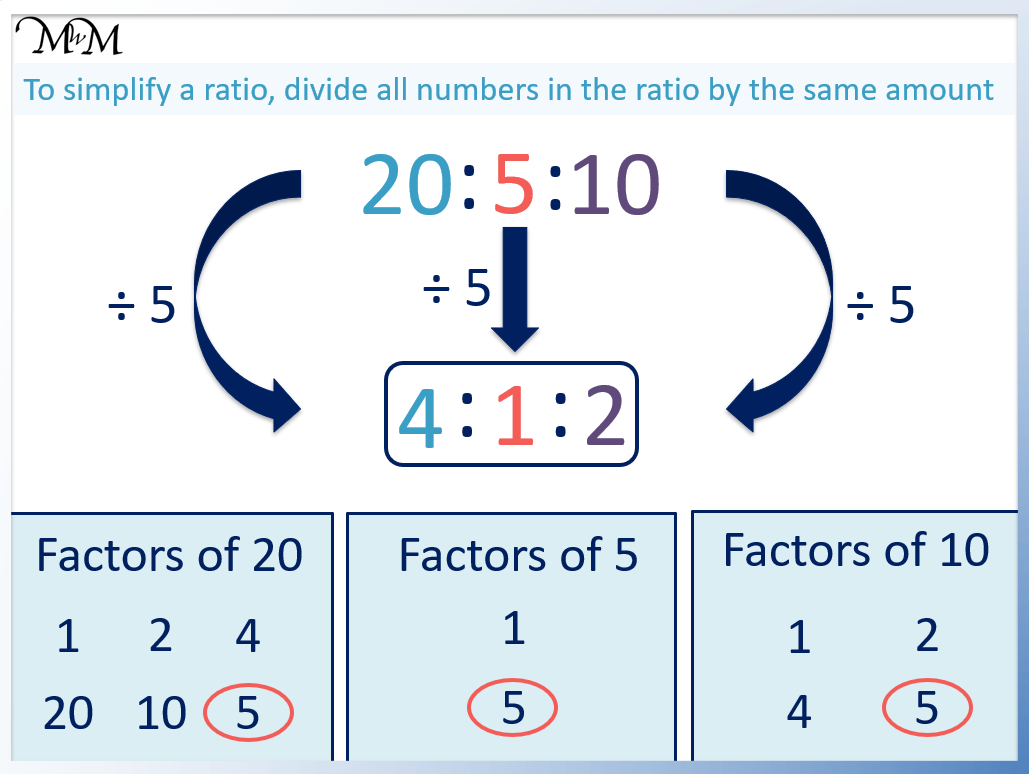Each of 20, 5 and 10 can be divided exactly by 5.

The ratio of 20:5:10 simplifies to 4:1:2.

It can help to list the factors of all 3 numbers before simplifying. Then to simplify in one go, divide all of the numbers by the largest number that is a factor of all of the numbers. This is called dividing by the highest common factor.

Here is another example of simplifying a ratio with 3 numbers.

Simplify the ratio of 8:12:4.The largest number that divides into each of 8, 12 and 4 is 4.

Dividing each of the numbers in 8:12:4 by 4, the ratio is simplified to 2:3:1.

## How to Simplify Ratios with Large Numbers

Large ratios can be simplified in steps. First, list the factors of each number. Then divide all of the numbers in the ratio by a number that is a factor of all of the numbers in the ratio. Keep repeating these steps until the numbers cannot be divided exactly anymore.

For example, simplify the ratio 200:150.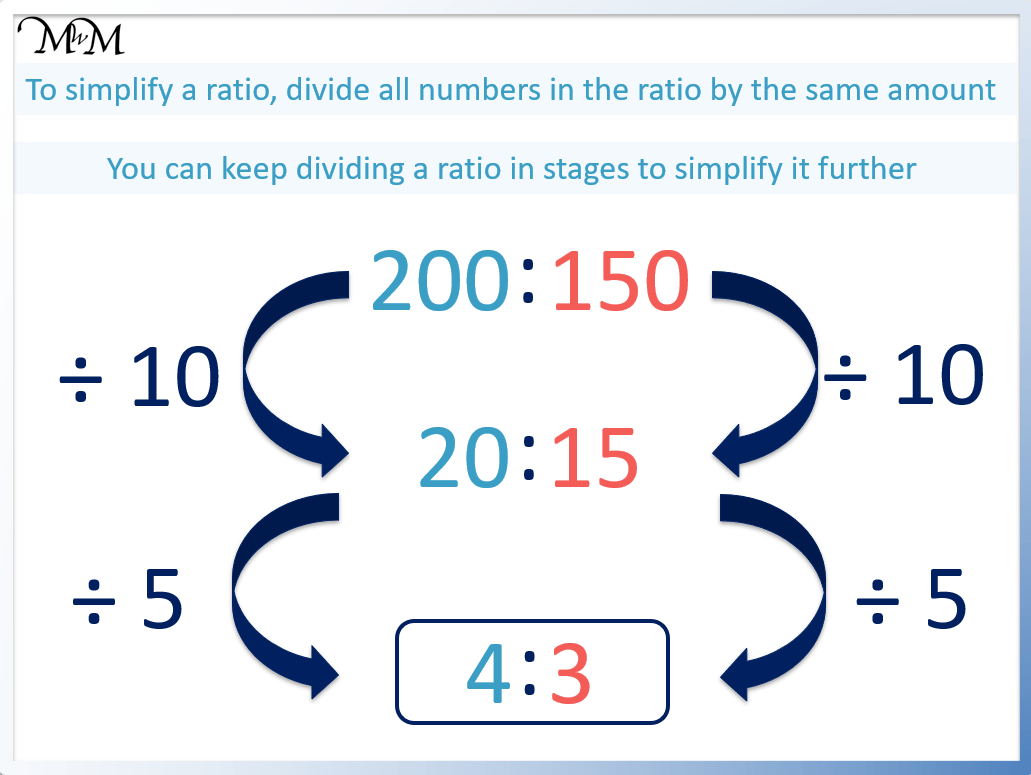The ratio will be simplified in steps.

We can see that both 200 and 150 end in a zero and so, they can both be divided exactly by 10.

This simplifies the ratio 200:150 to 20:15.

The ratio 20:15 can be simplified further by dividing by 5.

20:15 simplifies to 4:3.

200:150 is fully simplified as 4:3.

It is possible to simplify 200:150 to 4:3 in one step by dividing both numbers by 50. However, it can be easier to simplify the numbers in steps.

## How to Simplify a Ratio Involving Fractions

To simplify a ratio involving fractions:

1. Rewrite the fractions with equivalent fractions so that they all have the same denominator.
2. Write the numerators of these fractions as a ratio.
3. Simplify this ratio if possible by dividing by the highest common factor.

Simplify the ratio of the fractions 1/2 : 2/3.

1/2 and 2/3 have denominators of 2 and 3 respectively. Both fractions can be made into equivalent fractions with a denominator of 6.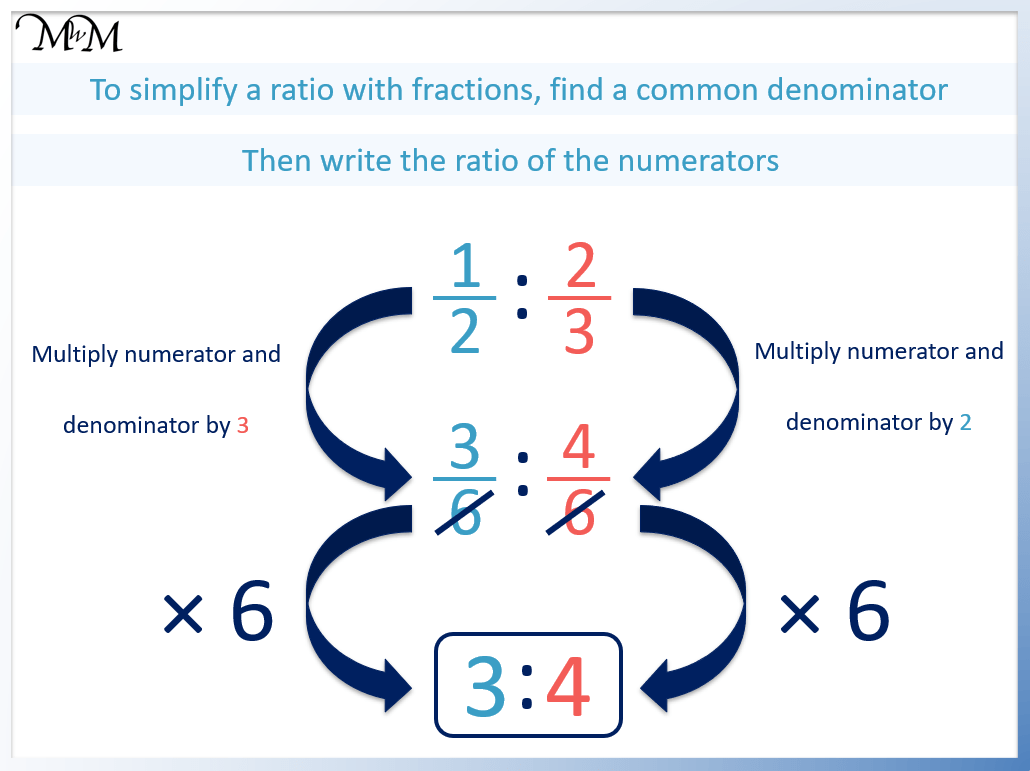1/2 can be written as 3/6 and 2/3 can be written as 4/6.

The next step is to write the numerators of the fractions as a ratio. Technically, both fractions are multiplied by 6 to remove the denominator.

The numerators are now 3 and 4 respectively and so, the ratio is 3:4.

There is a shortcut to simplifying ratios containing 2 fractions.

We can multiply the denominator of one fraction by the numerator of the other fraction.We multiply the denominator of 2/3 by the numerator of 1/2.

We multiply the denominator of 1/2 by the numerator of 2/3.

3 × 1 = 3 and 2 × 2 = 4.

The ratio is 3:4.

## How to Simplify a Ratio Involving Decimals

To simplify a ratio with decimals:

1. Multiply all of the numbers in the ratio by one of 10, 100, 1000 etc. so that the decimals are all whole numbers.
2. Simplify this ratio by dividing the numbers in the ratio by their highest common factor.

For example, simplify the ratio containing the decimals 0.2:1.6.Multiplying both decimals by 10 turns the ratio into 2:16. The ratio is now written with whole numbers.

Now the ratio can be simplified.

Dividing both numbers by 2, the ratio 2:16 simplifies to 1:8.

A ratio can contain decimal numbers. However, when a ratio is simplified, the decimal numbers are multiplied to make whole numbers. A simplified ratio should not contain decimal numbers.

Here is another example of simplifying decimal ratios.

Simplify the ratio of 0.5:0.15.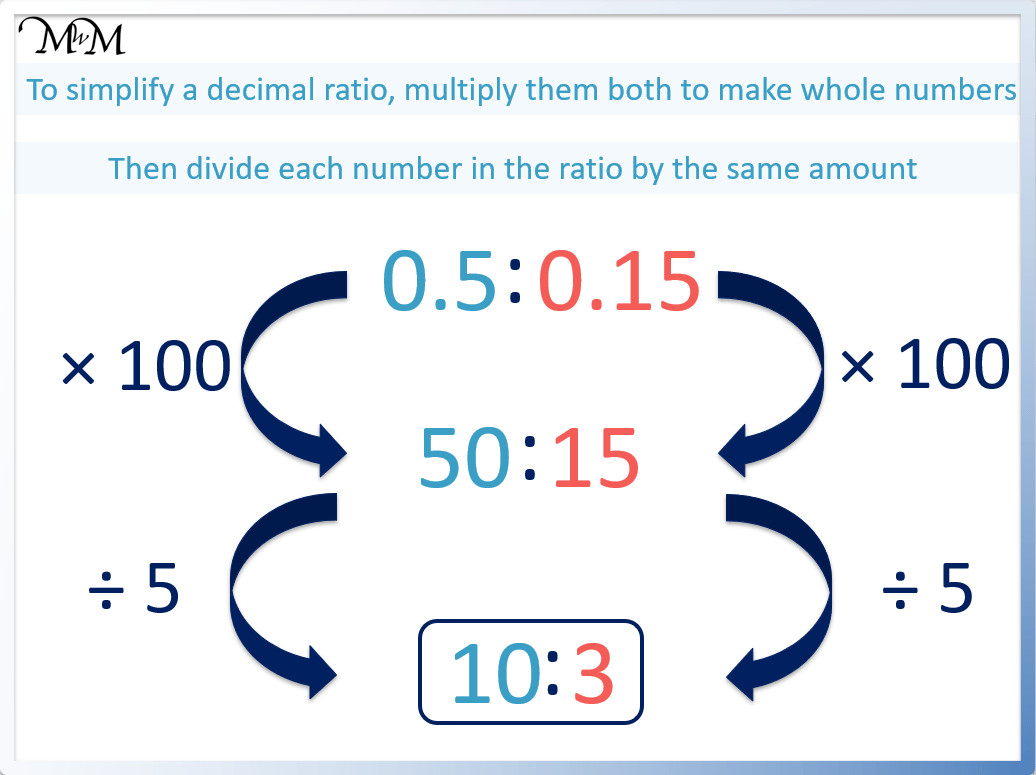Since 0.15 contains 2 decimal places, we multiply it by 100 to make it a whole number.

Multiplying both 0.5 and 0.15 by 100, the ratio becomes 50:15.

This ratio can be fully simplified by dividing both numbers by 5.

50:15 simplifies to 10:3.

## How to Simplify a Ratio with Different Units

To simplify ratios with different units:

1. Convert the larger unit to the smaller unit.
2. Simplify the ratio by dividing the numbers by their highest common factor

The numbers in a ratio must be converted to have the same units when they are simplified.

For example, simplify the ratio of 2 cm : 15 mm. We can see that one unit is centimetres and the other is millimetres.The first step is to convert the larger unit (centimetres) to the smaller unit (millimetres). We do this by multiplying by 10, since there are 10 mm in 1 cm.

2 cm: 15 mm becomes 20 mm : 15 mm.

This ratio can be simplified by dividing by 5.

20 mm: 15 mm becomes 4:3. Because the units are both the same (mm), we do not need to write them in our answer.

Here is another example of simplifying a ratio with different units.

Simplify 65 cm: 1m.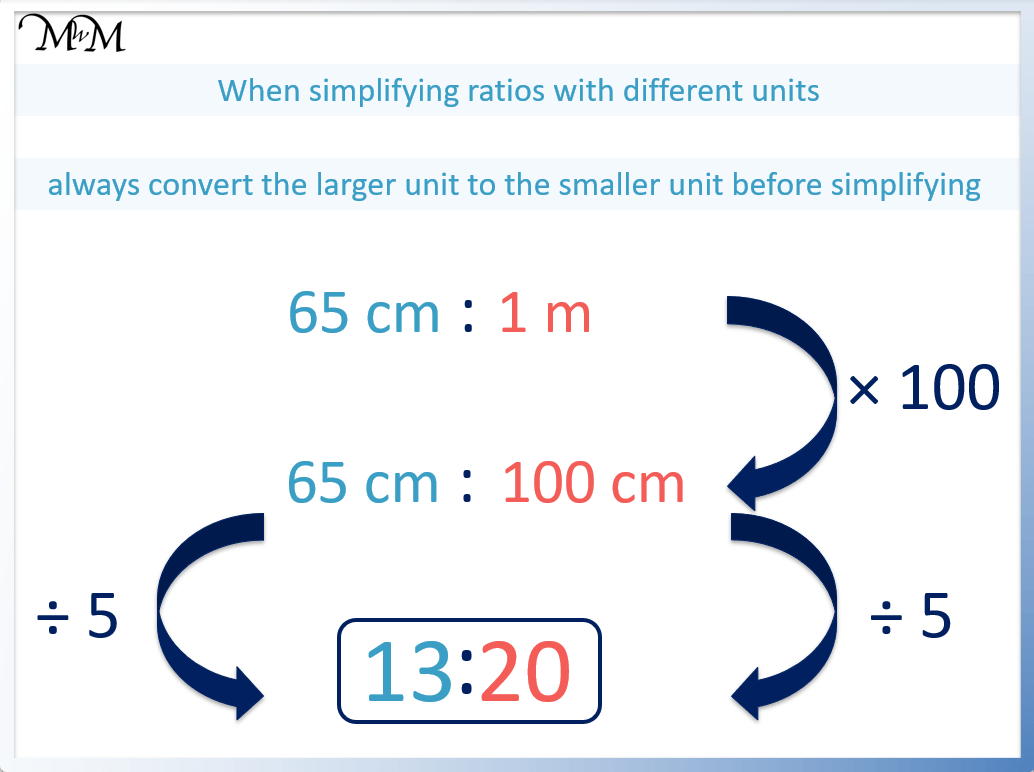We first convert metres to centimetres by multiplying by 100. There are 100 cm in 1 m.

65 cm: 1m becomes 65 cm : 100 cm.

We can now simplify by dividing by 5.

65 cm : 100 cm simplifies to 13:20.

Again we do not need to write the units because they are the same.

## Why Simplify a Ratio

Ratios are simplified to find smaller numbers that are easier to work with. Simplifying ratios may also remove any fractions or decimals that may be there. This results in a ratio that is easier to understand and do calculations with.Now try our lesson on How to Calculate a Ratio of 3 Numbers where we learn how to share an amount into a ratio of 3 parts.